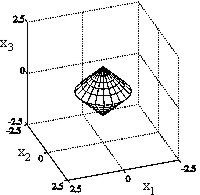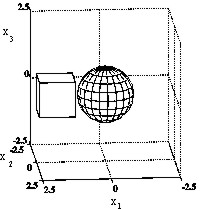# The use of optimal control methods to study two new classes of smart obstacles in time dependent acoustic scattering

## Abstract

Time dependent acoustic scattering problems involving "smart" obstacles are considered. Smart obstacles are obstacles that when hit by an incident acoustic field react in the attempt of pursuing a preassigned goal. Let IR3 be the three dimensional real Euclidean space and let W Ì IR3 be a bounded simply connected open set with Lipschitz boundary characterized by a constant acoustic boundary impedance c and immersed in an isotropic and homogeneous medium that fills IR3\W. The closure of W will be denoted with `W. When hit by an incident field the obstacle W pursues the preassigned goal circulating on its boundary a suitable pressure current (i.e. a quantity dimensionally given by a pressure divided by a time). The obstacles considered in this paper choose the pressure current to circulate on their boundaries in order to fulfill one of the following goals: i) to be furtive in a given set of the frequency space, ii) to appear in a given set of the frequency space and outside a given set of IR3 containing W and WG as similar as possible to a "ghost" obstacle WG having boundary acoustic impedance cG. We assume that `WÇ`WG = Æ and that WG ¹ Æ. The problem corresponding to the first goal will be called definite band furtivity problem, the problem corresponding to the second goal will be called definite band ghost obstacle problem. These goals define two classes of smart obstacles. Let t denote the time variable, the main purpose of this paper is to model the previous problems as optimal control problems for the wave equation introducing a control function (i.e. the pressure current) acting on the boundary of W for t Î IR. The cost functionals proposed depend on the value of the control function on the boundary of the obstacle and on the value of the scattered acoustic field generated by the obstacle on the boundary of the obstacle (in the "furtivity case") or on the boundary of a suitable set containing W and WG (in the "ghost obstacle case"). Under some hypotheses the use of the Pontryagin maximum principle allows us to formulate the first order optimality conditions for the definite band furtivity problem and for the definite band ghost obstacle problem as exterior problems outside the obstacle for a system of two coupled wave equations. Numerical methods to solve these exterior problems are developed. These methods are adapted versions of the method introduced in , , , they belong to the class of the operator expansion methods and are highly parallelizable. Some numerical experiments proving the validity of the control problems proposed as mathematical models of the definite band furtivity problem and of the definite band ghost obstacle problem are shown. The numerical results obtained with a parallel implementation of the numerical methods developed are discussed and their quantitative character is established. The speed up factors obtained using parallel computing are really impressive. This website contains some animations and some virtual reality applications relative to the numerical experiments.

## References


E. Mecocci, L. Misici, M. C. Recchioni and F. Zirilli, "A new formalism for time dependent wave scattering from a bounded obstacle", Journal of the Acoustical Society of America 107, 1825-1840 (2000)
.

F. Mariani, M.C. Recchioni and F. Zirilli, "The use of the Pontryagin maximum principle in a furtivity problem in time-dependent acoustic obstacle scattering", Waves in Random Media 11, 549-575 (2001).

L. Fatone, M. C. Recchioni and F. Zirilli, "A masking problem in time dependent acoustic obstacle scattering", ARLO - {Acoustics Research Letters Online}, 5, Issue 2, 25-30 (2004).

Warning: To see the virtual reality animations we recommend to use at least the following resources:
```- screen resolution: 1024 x 768
- processor: Pentium II or equivalent
```

We suggest the following VRML clients:

 Windows Mac UNIX/Linux Cortona X X Cosmo Player X X OpenVRML (Under Developement) X FreeWRL (Under Developement) X

Note that in a Unix/Linux environment the clients OpenVRML and FreeWRL allow the visualization of virtual objects but not the visualization of virtual scenes.

Definite Band Furtivity Problem: Double ConeDouble Cone (virtual object): VRML visualization

Remark. In the following animations the time values shown in the twenty frames are t=-2+0.25j, j=0,1,...,19.

Furtivity effect in the assigned frequency band Data: incident wave ui=exp(-(z+t)2), band K=(-1, 1), scatterer double cone Animation 1: Double Cone (gif file)

Furtivity effect outside the assigned frequency band Data: incident wave ui=exp(-(z+t)2), band K=(-1, 1), scatterer double cone Animation 2: Double Cone (gif file)

Definite Band Ghost Problem: Cube-SphereCube-Sphere (virtual objects): VRML visualization

Remark. In the following animations the scattered fields that is, the field scattered by the passive obstacle, the field scattered by the active obstacle and the field scattered by the ghost, are multiplied by 2. The time values shown in the twenty frames of the animations are t=-3+0.75j, j=0,1,...,19.

Ghost effect in the assigned frequency band: Data: incident wave ui=exp(-(x-t)2), band K=(-∞, +∞), scatterer sphere, ghost cube Animation 3: Cube-Sphere (Virtual scene: VRML file)

Ghost effect in the assigned frequency band: The Animation 4 shows on the surface of the sphere of center the origin and radius 3 the convolution of the inverse Fourier transform of the characteristic function of the set K with the wave scattered by the passive obstacle, with the wave scattered by active obstacle and with the wave scattered by the ghost respectively in a colour The x-axis represents the spherical coordinate variable f, 0£f£2p and the y-axis represents the spherical coordinate variable q, 0£q£p . Data: incident wave ui=exp(-(x-t)2), band K=(-1.5, 1.5), scatterer sphere, ghost cube Animation 4: Ghost Effect on the definite band K=(-1.5,1.5) (mpeg file)

Ghost effect outside the assigned frequency band: The Animation 5 shows on the surface of the sphere of center the origin and radius 3 the convolution of the inverse Fourier transform of the characteristic function of the set IR\K with the wave scattered by the passive obstacle, with the wave scattered by active obstacle and with the wave scattered by the ghost respectively in a colour The x-axis represents the spherical coordinate variable f, 0£f£2p and the y-axis represents the spherical coordinate variable q, 0£q£p . Data: incident wave ui=exp(-(x-t)2), band K=(-1.5, 1.5), scatterer sphere, ghost cube Animation 5: Ghost Effect outside of the definite band K=(-1.5,1.5) (mpeg file)

Entry n.